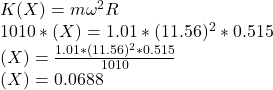## A 1.01 kg mass is attached to a spring of force constant 10.1 N/cm and placed on a frictionless surface. By how much will the spring stretch

Question

A 1.01 kg mass is attached to a spring of force constant 10.1 N/cm and placed on a frictionless surface. By how much will the spring stretch if the mass moves along a circular path of radius 0.515 m at a rate of 1.84 revolutions per second

in progress 0
7 months 2021-07-27T11:43:02+00:00 1 Answers 3 views 0

6.88 cm

Explanation:

Given that:

mass m of the object  = 1.0 kg

Force constant of the spring (k) = 10.1 N/cm = 10.1 × (100) N/m

= 1010 N/m

Radius of the circular path R = 0.515 m

Since 1 revolution = 2 π radian

angular speed of the object ω  = 1.84 rev/s = 1.84 × ( 2 π) rad/s